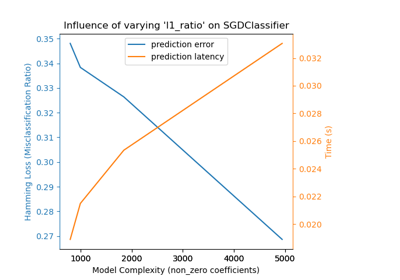# sklearn.metrics.hamming_loss¶

sklearn.metrics.hamming_loss(y_true, y_pred, labels=None, sample_weight=None)[source]

Compute the average Hamming loss.

The Hamming loss is the fraction of labels that are incorrectly predicted.

Read more in the User Guide.

Parameters: y_true : 1d array-like, or label indicator array / sparse matrix Ground truth (correct) labels. y_pred : 1d array-like, or label indicator array / sparse matrix Predicted labels, as returned by a classifier. labels : array, shape = [n_labels], optional (default=’deprecated’) Integer array of labels. If not provided, labels will be inferred from y_true and y_pred. New in version 0.18. Deprecated since version 0.21: This parameter labels is deprecated in version 0.21 and will be removed in version 0.23. Hamming loss uses y_true.shape for the number of labels when y_true is binary label indicators, so it is unnecessary for the user to specify. sample_weight : array-like of shape = [n_samples], optional Sample weights. New in version 0.18. loss : float or int, Return the average Hamming loss between element of y_true and y_pred.

Notes

In multiclass classification, the Hamming loss corresponds to the Hamming distance between y_true and y_pred which is equivalent to the subset zero_one_loss function, when normalize parameter is set to True.

In multilabel classification, the Hamming loss is different from the subset zero-one loss. The zero-one loss considers the entire set of labels for a given sample incorrect if it does not entirely match the true set of labels. Hamming loss is more forgiving in that it penalizes only the individual labels.

The Hamming loss is upperbounded by the subset zero-one loss, when normalize parameter is set to True. It is always between 0 and 1, lower being better.

References

  Grigorios Tsoumakas, Ioannis Katakis. Multi-Label Classification: An Overview. International Journal of Data Warehousing & Mining, 3(3), 1-13, July-September 2007.

Examples

>>> from sklearn.metrics import hamming_loss
>>> y_pred = [1, 2, 3, 4]
>>> y_true = [2, 2, 3, 4]
>>> hamming_loss(y_true, y_pred)
0.25


In the multilabel case with binary label indicators:

>>> import numpy as np
>>> hamming_loss(np.array([[0, 1], [1, 1]]), np.zeros((2, 2)))
0.75


## Examples using sklearn.metrics.hamming_loss¶Model Complexity Influence